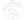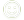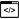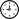Courses Details
Artificial Intelligence & Deep Learning Course with TensorflowCourse Code AI-TensorflowDuration 5 Days
Request Group Training
Course Overview
Artificial Intelligence Deep Learning with TensorFlow course is an industry-designed certification training to master Convolutional Neural Network (CNN), Perceptron in CNN, TensorFlow, TensorFlow-Code, graph visualization, transfer learning, recurrent neural networks, deep learning libraries, Keras & TFLearn API, GPU in deep learning, backpropagation, and hyperparameters through hands-on projects. Master AI in this Artificial Intelligence Deep Learning certification course.

Artificial Intelligence today is taking over each industry domain. Machine Learning and especially Deep Learning are the most important aspects of Artificial Intelligence that are being deployed everywhere from search engines to online movie recommendations. Taking the Deep Learning training can help professionals to build a solid career in a rising technology domain and get the best jobs in top organizations.
Course Schedule
Target Audience
Professionals in analytics, data science domains, ecommerce, search engine domains
Software professionals looking for a career switch and fresh graduates
Course Prerequisites
Basic IT skills , experience with python
Expected Accomplishments
At the end of the course you will be able to
• Fundamentals of Deep Learning techniques
• Understanding an Artificial Neural Network
• How to train a neural network with training data
• Convolutional neural networks and its applications
• TensorFlow and Tensor Processing Unit
• Supervised and unsupervised learning methodology
• Machine learning with Python language
• Application of DL in image recognition, NLP and more
• Real world projects in recommender systems and others
Course Outline
Introduction to Deep Learning & Neural Networks
The domain of machine learning and its implications to the artificial intelligence sector, the advantages of machine learning over other conventional methodologies, introduction to Deep Learning within machine learning, how it differs from all others methods of machine learning, training the system with training data, supervised and unsupervised learning, classification and regression supervised learning, clustering and association unsupervised learning, the algorithms used in these types of learning. Introduction to AI, Introduction to Neural Networks, Supervised Learning with Neural Networks, Concept of Machine Learning, Basics of statistics, probability distributions, hypothesis testing, Hidden Markov Model.

Multi-layered Neural Networks
Introduction to Multi Layer Network, Concept of Deep neural networks, Regularization. Multi-layer perceptron, capacity and overfitting, neural network hyperparameters, logic gates, the various activation functions in neural networks like Sigmoid, ReLu and Softmax, hyperbolic functions. Backpropagation, convergence, forward propagation, overfitting, hyperparameters.

Training of neural networks
The various techniques used in training of artificial neural networks, gradient descent rule, perceptron learning rule, tuning learning rate, stochastic process, optimization techniques, regularization techniques, regression techniques Lasso L1, Ridge L2, vanishing gradients, transfer learning, unsupervised pre-training, Xavier initialization, vanishing gradients.

Deep Learning Libraries
How Deep Learning Works, Activation Functions, Illustrate Perceptron, Training a Perceptron, Important Parameters of Perceptron, Multi-layer Perceptron What is TensorFlow, Introduction to TensorFlow open source software library for designing, building and training Deep Learning models, Python Library behind TensorFlow, Tensor Processing Unit (TPU) programmable AI accelerator by Google, TensorFlow code-basics, Graph Visualization, Constants, Placeholders, Variables, Step by Step – Use-Case Implementation, Keras.

Introduction to Keras API
Keras high-level neural network for working on top of TensorFlow, defining complex multi-output models, composing models using Keras, sequential and functional composition, batch normalization, deploying Keras with TensorBoard, neural network training process customization.
TFLearn API for TensorFlow
Implementing neural networks using TFLearn API, defining and composing models using TFLearn, deploying TensorBoard with TFLearn.

DNN: Deep Neural Networks
Mapping the human mind with Deep Neural Networks, the various building blocks of Artificial Neural Networks, the architecture of DNN, its building blocks, the concept of reinforcement learning in DNN, the various parameters, layers, activation functions and optimization algorithms in DNN.

CNN: Convolutional Neural Networks
What is a Convolutional Neural Network, understanding the architecture of CNN, use cases of CNN, what is a pooling layer, how to visualize using CNN, how to fine-tune a Convolutional Neural Network, what is Transfer Learning and understanding Recurrent Neural Networks, feature maps, Kernel filter, pooling, deploying convolutional neural network in TensorFlow

RNN: Recurrent Neural Networks
Intro to RNN Model, Application use cases of RNN, Modelling sequences, Training RNNs with Backpropagation, Long Short-Term memory (LSTM), Recursive Neural Tensor Network Theory, Recurrent Neural Network Model, basic RNN cell, unfolded RNN, training of RNN, dynamic RNN, time-series predictions.

GPU in Deep Learning
Introduction to GPUs and how they differ from CPUs, the importance of GPUs in training Deep Learning Networks, the forward pass and backward pass training technique, the GPU constituent with simpler core and concurrent hardware.

Autoencoders & Restricted Boltzmann Machine (RBM)
Introduction to RBM and autoencoders, deploying it for deep neural networks, collaborative filtering using RBM, features of autoencoders, applications of autoencoders.

Chatbots
Automated conversation bots using one of the descriptive techniques
IBM Watson